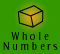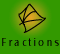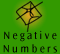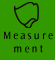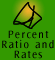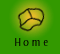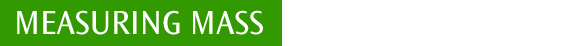| What is mass? | Mass measurement facts|
| Quick quiz |

What is mass?

Mass is the quantity of matter contained in an object. My car has a mass of 800 kilograms. Strictly speaking although we often refer to weight when we mean mass, weight is a force that is exerted by the gravitational pull of the Earth on an object.

For example, an astronaut who had a mass of 90 kgs on Earth would still have a mass of 90 kgs in space, but he would have no or very little weight due to the lack of gravitational pull.

In every day conditions on Earth we often take the mass and weight to mean the same thing.

Because it is difficult to give a definition of mass, it is imperative that students undertake structured exploration of objects: (i) that have the same mass but differ on other attributes (especially volume) and (ii) that have different mass but are the same on other attributes.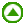Mass measurement Facts

 MASS SI unit: kilogram (kg) 1000 milligrams (mg) = 1 gram (g) 1000 grams (g) = 1 kilogram (kg) 1000 kilograms (kg) = 1 megagram (Mg) = 1 tonne

Example 1: The mass of an average sized car is 1000 kilograms. What is the mass in tonnes of 25 average sized cars?

 Working Out Thinking 1 car weighs 1000 kg 25 cars weigh 25 x 1000 kg The mass of 25 average cars is 25000 kg 25000 kg = 25 tonnes The mass of 25 average cars is 25 tonnes I know 1 car has a mass of 1000 kg, therefore 25 cars will have a mass of 25000 kg. I now have to convert kilograms to tonnes. There are 1000 kg in a tonne, and I am converting from a smaller unit to a larger unit, so I need to divide my answer by 1000 to express it in tonnes.

Example 2: Harold went on a diet which guaranteed that if he kept to the program he would lose on average 500 grams per week. If, after 7 weeks, Harold lost 3.7 kilograms, did he achieve the guaranteed weight loss?

 Working Out Thinking 7 x 500 g = 3500 g   3.7 kg = 3700 g 3700 g > 3500 g Therefore Harold lost more weight than was guaranteed by the diet as he had achieved the guaranteed weight loss. First I need to work out how much weight Harold should have lost according to the diet in the 7 week period. At 500 gs per week this makes 3500 gs in 7 weeks. He actually lost 3.7 kgs which is equivalent to 3700 gs, so he lost more weight than the diet guaranteed.Quick quiz

 1. a) How many kilograms are there in one tonne? b) What is the mass of one millilitre of water? c) How many milligrams are in one gram? d) How many milligrams are in one kilogram? 2. What are the most appropriate units to use when measuring the mass of the following objects? a) car b) book c) computer d) person e) a cup of flour f) feather 3. What is the difference between weight and mass? 4. a) I need to add 150 grams of flour to 100 grams of coconut and 200 grams of sugar. I then add 300 grams of mixed dried fruit. What is the total mass of these uncooked ingredients? b) If there are 25 students in your class and they have an average weight of 45 kilograms, what is the estimated total mass of your class in (i) kilograms and (ii) tonnes?